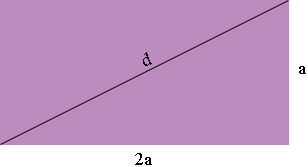SEARCH HOMEMath Central Quandaries & QueriesQuestion from Ann, a student: given a rectangle with sides a and 2a and diagonal d, find the area of the rectangle as a function of the diagonal,dHi Ann,Since the area of a rectangle is the length times the height the area of this rectangle is

area = 2a × a = 2a2.

Look at the diagram. Do you see a right triangle? What does Pythagoras tell you? Use this relationship to write the area of the rectangle in terms if d.

PennyMath Central is supported by the University of Regina and The Pacific Institute for the Mathematical Sciences.